# How to use i.MX RT1060 to classify the clothing

cancel
Showing results for
Did you mean:

# How to use i.MX RT1060 to classify the clothing

## How to use i.MX RT1060 to classify the clothing

In the tutorial, I'd like to show the steps of deploying an image classification model on i.MX RT1060 to enabling you to classify fashion images and categories.
In the first part of this tutorial, we will review the Fashion MNIST dataset, including how to download it to your system. From there we’ll define a simple CNN network using the TensorFlow platform.
Next, we’ll train our CNN model on the Fashion MNIST dataset, train it, and review the results.
Finally, we'll optimize the model, after that, the model will be smaller and increase inferencing speed, which is valuable for source-limited devices such as MCU.
Let’s go ahead and get started!

# Fashion MNIST dataset

The Fashion MNIST dataset was created by the e-commerce company, Zalando.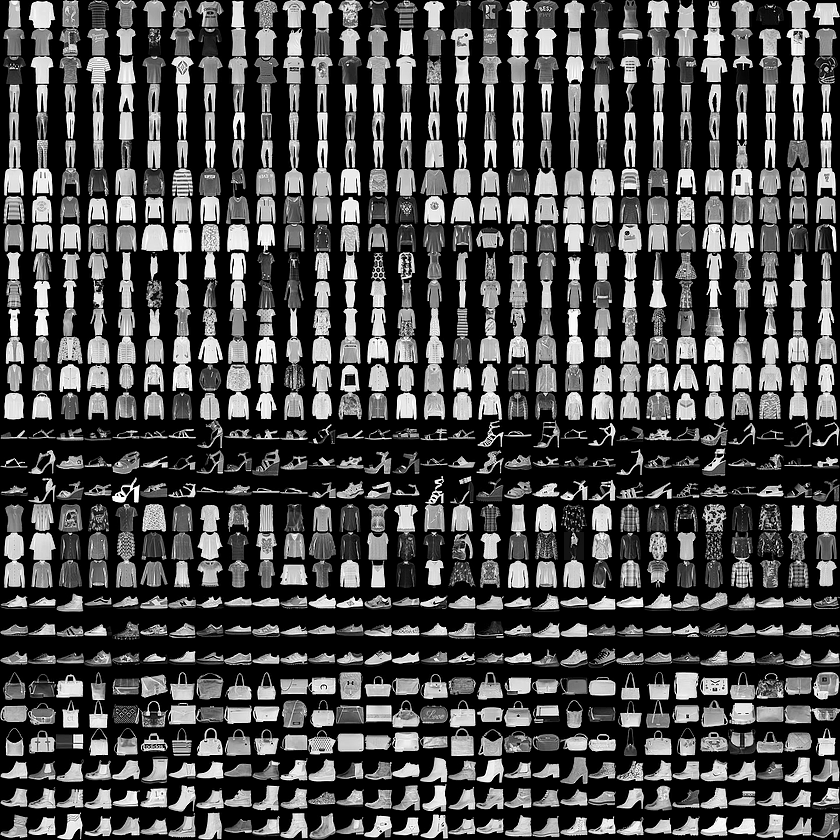Fig 1 Fashion MNIST dataset

As they note on their official GitHub repo for the Fashion MNIST dataset, there are a few problems with the standard MNIST digit recognition dataset:

1. It’s far too easy for standard machine learning algorithms to obtain 97%+ accuracy.
2. It’s even easier for deep learning models to achieve 99%+ accuracy.
3. The dataset is overused.
4. MNIST cannot represent modern computer vision tasks.

Zalando, therefore, created the Fashion MNIST dataset as a drop-in replacement for MNIST.

• 60,000 training examples
• 10,000 testing examples
• 10 classes: T-shirt/top, Trouser, Pullover, Dress, Coat, Sandal, Shirt, Sneaker, Bag, Ankle boot
• 28×28 grayscale images

The code below loads the Fashion-MNIST dataset using the TensorFlow and creates a plot of the first 25 images in the training dataset.

``````import tensorflow as tf
import numpy as np
# For easy reset of notebook state.
tf.keras.backend.clear_session()
fashion_mnist = tf.keras.datasets.fashion_mnist

(train_images, train_labels), (test_images, test_labels) = fashion_mnist.load_data()

lass_names = ['T-shirt/top', 'Trouser', 'Pullover', 'Dress', 'Coat',
'Sandal', 'Shirt', 'Sneaker', 'Bag', 'Ankle boot']

plt.figure(figsize=(8,8))
for i in range(25):
plt.subplot(5,5,i+1,)
plt.tight_layout()
plt.imshow(train_images[i])
plt.xlabel(lass_names[train_labels[i]])
plt.xticks([])
plt.yticks([])
plt.grid(False)
plt.show()``````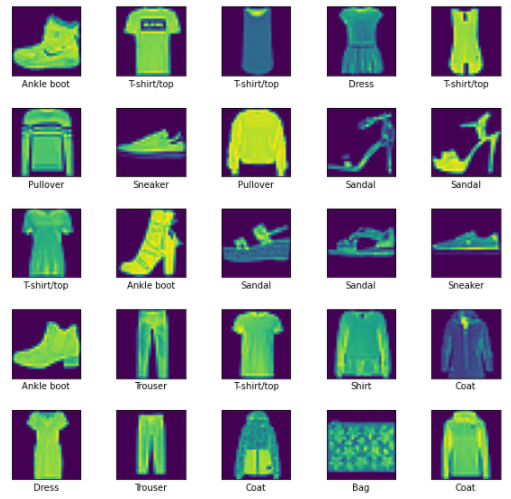Fig 2

Running the code loads the Fashion-MNIST train and test dataset and prints their shape.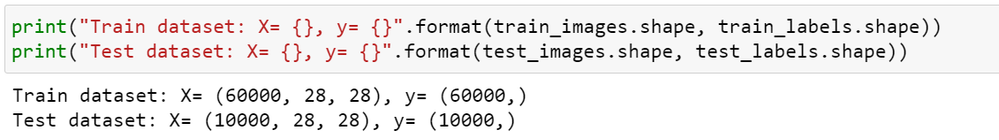Fig 3

We can see that there are 60,000 examples in the training dataset and 10,000 in the test dataset and that images are indeed square with 28×28 pixels.

# Creating model

We need to define a neural network model for the image classify purpose, and the model should have two main parts: the feature extraction and the classifier that makes a prediction.

## Defining a simple Convolutional Neural Network (CNN)

For the convolutional front-end, we build 3 layers of convolution layer with a small filter size (3,3) and a modest number of filters followed by a max-pooling layer. The last filter map is flattened to provide features to the classifier.
As we know, it's a multi-class classification task, so we will require an output layer with 10 nodes in order to predict the probability distribution of an image belonging to each of the 10 classes. In this case, we will require the use of a softmax activation function. And between the feature extractor and the output layer, we can add a dense layer to interpret the features.
All layers will use the ReLU activation function and the He weight initialization scheme, both best practices.
We will use the Adam optimizer to optimize the sparse_categorical_crossentropy loss function, suitable for multi-class classification, and we will monitor the classification accuracy metric, which is appropriate given we have the same number of examples in each of the 10 classes.
The below code will define and run it will show the struct of the model.

``````# Define a Model
model =  tf.keras.models.Sequential()
# First Convolution ，Kernel:16*3*3
model.add( tf.keras.layers.Conv2D(16, (3, 3), activation='relu', kernel_initializer='he_uniform',input_shape=(28, 28, 1)))
# Second Convolution ，Kernel:32*3*3
# Third Convolution ，Kernel:32*3*3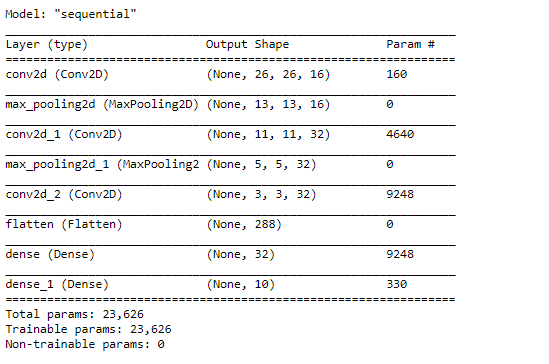Fig 4

## Training Model

After the model is defined, we need to train it.
The model will be trained using 5-fold cross-validation. The value of k=5 was chosen to provide a baseline for both repeated evaluation and to not be too large as to require a long running time. Each validation set will be 20% of the training dataset or about 12,000 examples.
The training dataset is shuffled prior to being split and the sample shuffling is performed each time so that any model we train will have the same train and validation datasets in each fold, providing an apples-to-apples comparison.
We will train the baseline model for a modest 20 training epochs with a default batch size of 32 examples. The validation set for each fold will be used to validate the model during each epoch of the training run, so we can later create learning curves, and at the end of the run, we use the test dataset to estimate the performance of the model. As such, we will keep track of the resulting history from each run, as well as the classification accuracy of the fold.
The train_model() function below implements these behaviors, taking the training dataset and test dataset as arguments, and returning a list of accuracy scores and training histories that can be later summarized.

``````from sklearn.model_selection import KFold

# train a model using k-fold cross-validation
def train_model(dataX, dataY, n_folds=5):
scores, histories = list(), list()
# prepare cross validation
kfold = KFold(n_folds, shuffle=True, random_state=1)

for train_ix, validate_ix in kfold.split(dataX):
# select rows for train and test
trainX, trainY, validate_X, validate_Y = dataX[train_ix], dataY[train_ix], dataX[validate_ix], dataY[validate_ix]
# fit model
history = model.fit(trainX, trainY, epochs=20, batch_size=32, validation_data=(validate_X, validate_Y), verbose=0)
# evaluate model
_, acc = model.evaluate(validate_X, validate_Y, verbose=0)
print("Accurary: {:.4f}，Total number of figures is {:0>2d}".format(acc * 100.0, len(testY)))
# append scores
scores.append(acc)
histories.append(history)
return scores, histories``````

## Module Summary

After the model has been trained, we can present the results.
There are two key aspects to present: the diagnostics of the learning behavior of the model during training and the estimation of the model performance. These can be implemented using separate functions.

First, the diagnostics involve creating a line plot showing model performance on the train and validate set during each fold of the k-fold cross-validation. These plots are valuable for getting an idea of whether a model is overfitting, underfitting, or has a good fit for the dataset.

We will create a single figure with two subplots, one for loss and one for accuracy. Blue lines will indicate model performance on the training dataset and orange lines will indicate performance on the hold-out validate dataset. The summarize_diagnostics() function below creates and shows this plot given the collected training histories.

``````# plot diagnostic learning curves
def summarize_diagnostics(histories):
for i in range(len(histories)):
# plot loss
plt.subplot(2,1,1)
plt.title('Cross Entropy Loss')
plt.plot(histories[i].history['loss'], color='blue', label='train')
plt.plot(histories[i].history['val_loss'], color='orange', label='test')
# plot accuracy
plt.subplot(2,1,2)
plt.title('Classification Accuracy')
plt.plot(histories[i].history['accuracy'], color='blue', label='train')
plt.plot(histories[i].history['val_accuracy'], color='orange', label='test')
plt.show()``````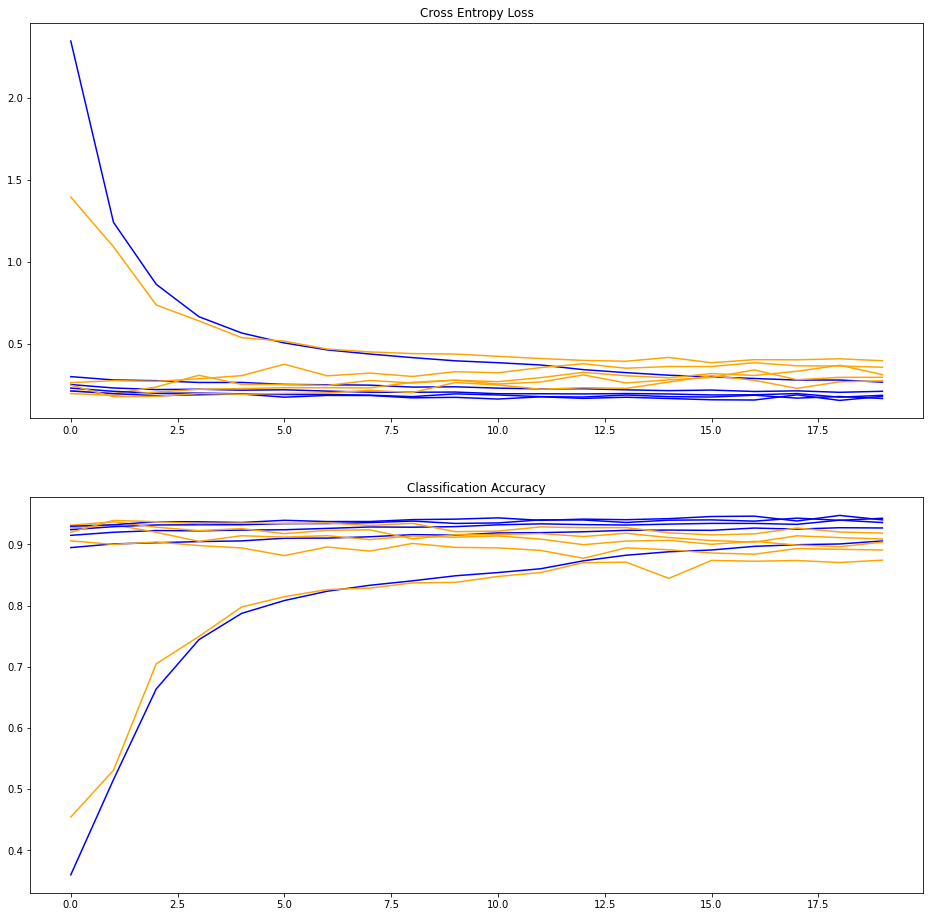Fig 5

Next, the classification accuracy scores collected during each fold can be summarized by calculating the mean and standard deviation. This provides an estimate of the average expected performance of the model trained on the test dataset, with an estimate of the average variance in the mean. We will also summarize the distribution of scores by creating and showing a box and whisker plot.
The summarize_performance() function below implements this for a given list of scores collected during model training.

``````# summarize model performance
def summarize_performance(scores):
# print summary
print('Accuracy: mean={:.4f} std={:.4f}, n={:0>2d}'.format(np.mean(trained_scores)*100, np.std(trained_scores)*100, len(scores)))
# box and whisker plots of results
plt.boxplot(scores)
plt.show()``````Fig 6

## Verifying predictions

According to the above figure, we see that the final trained model can get up to around 87.6% accuracy when predicting the test dataset.
And with the trained model, running the below code will demonstrate the result of predictions about some images.

``````def plot_image(i, predictions_array, true_label, img):
true_label, img = true_label[i], img[i]
plt.grid(False)
plt.xticks([])
plt.yticks([])

plt.imshow(img.reshape(28, 28), cmap=plt.cm.binary)

predicted_label = np.argmax(predictions_array)
if predicted_label == true_label:
color = 'blue'
else:
color = 'red'

plt.xlabel("{} {:2.0f}% ({})".format(class_names[predicted_label],
100*np.max(predictions_array),
class_names[true_label]),
color=color)

def plot_value_array(i, predictions_array, true_label):
true_label = true_label[i]
plt.grid(False)
plt.xticks(range(10))
plt.yticks([])
thisplot = plt.bar(range(10), predictions_array, color="#777777")
plt.ylim([0, 1])
predicted_label = np.argmax(predictions_array)

thisplot[predicted_label].set_color('red')
thisplot[true_label].set_color('blue')

predictions = model.predict(test_images)

# Plot the first X test images, their predicted labels, and the true labels.
# Color correct predictions in blue and incorrect predictions in red.
num_rows = 5
num_cols = 3
num_images = num_rows*num_cols
plt.figure(figsize=(2*2*num_cols, 2*num_rows))
for i in range(num_images):
plt.subplot(num_rows, 2*num_cols, 2*i+1)
plot_image(i, predictions[i], test_labels, test_images)
plt.subplot(num_rows, 2*num_cols, 2*i+2)
plot_value_array(i, predictions[i], test_labels)
plt.tight_layout()
plt.show()``````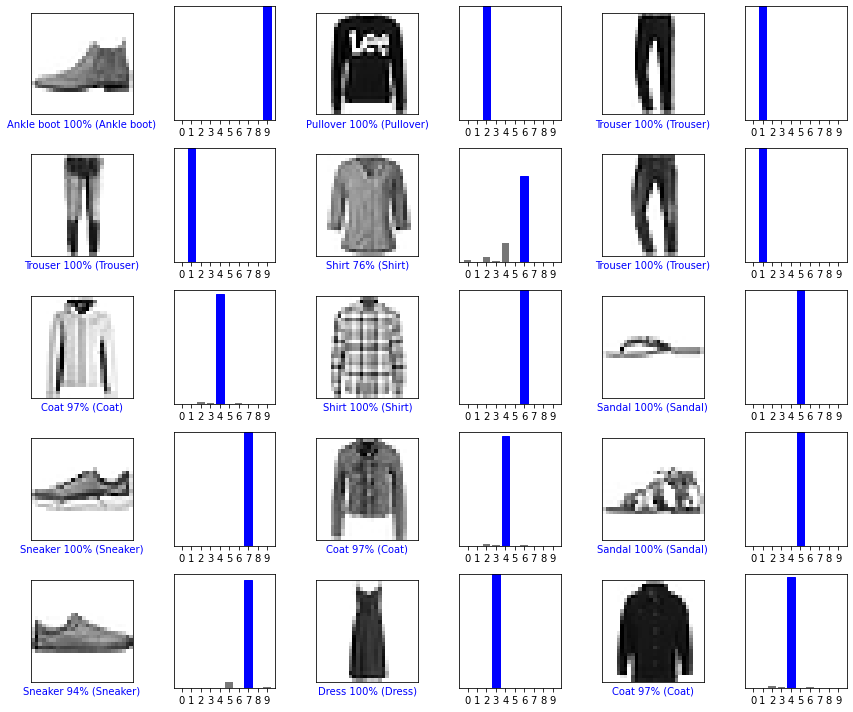Fig 7

# Model quantization

Post-training quantization is a conversion technique that can reduce model size while also improving CPU and hardware accelerator latency, with little degradation in model accuracy, especially it's crucial to embedded platforms, as it lacks the compute-intensive performance, the Flash and RAM memory is also very limited.
TensorFlow Lite is able to be used to convert an already-trained float TensorFlow model to the TensorFlow Lite format. In addition, the TensorFlow Lite provides several approaches to optimize the mode, among these ways, Integer quantization is an optimization strategy that converts 32-bit floating-point numbers (such as weights and activation outputs) to the nearest 8-bit fixed-point numbers. This results in a smaller model and increased inferencing speed, which is very valuable for low-power devices such as microcontrollers.
The below codes show how to implement the Integer quantization of the trained model, and after running these codes, we can find that the size of Tensorflow Lite mode reduces almost 64.9 KB versus the original model, becomes about 32% of the original size(Fig 8).

``````import os
# Convert using integer-only quantization
def representative_data_gen():
for input_value in tf.data.Dataset.from_tensor_slices(tf.cast(train_images,tf.float32)).shuffle(500).batch(1).take(150):
yield [input_value]

# Convert using dynamic range quantization
converter = tf.lite.TFLiteConverter.from_keras_model(model)
converter.optimizations = [tf.lite.Optimize.DEFAULT]

tflite_model_quant = converter.convert()

# Save the model to disk
open("model_dynamic_range_quantization.tflite", "wb").write(tflite_model_quant)
## Size difference
Dynamic_range_quantization_model_size = os.path.getsize("model_dynamic_range_quantization.tflite")
print("Dynamic range quantization model is %d bytes" % Dynamic_range_quantization_model_size)

converter = tf.lite.TFLiteConverter.from_keras_model(model)
converter.optimizations = [tf.lite.Optimize.DEFAULT]
converter.representative_dataset = representative_data_gen
# Ensure that if any ops can't be quantized, the converter throws an error
converter.target_spec.supported_ops = [tf.lite.OpsSet.TFLITE_BUILTINS_INT8]
# Set the input and output tensors to uint8 (APIs added in r2.3)
converter.inference_input_type = tf.uint8
converter.inference_output_type = tf.uint8

# Save the model to disk

Integer_only_quantization_model_size = os.path.getsize("model_integer_only_quantization.tflite")
print("Integer_only_quantization_model is %d bytes" % Integer_only_quantization_model_size)
difference = Dynamic_range_quantization_model_size - Integer_only_quantization_model_size
print("Difference is %d bytes" % difference)``````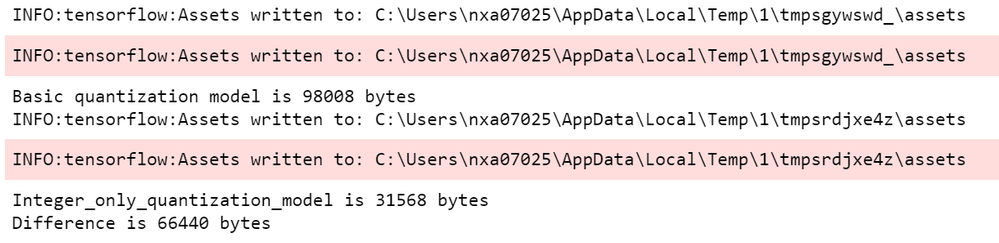Fig 8

# Evaluating the TensorFlow Lite model

Now we'll run inferences using the TensorFlow Lite Interpreter to compare the model accuracies.
First, we need a function that runs inference with a given model and images, and then returns the predictions:

``````# Helper function to run inference on a TFLite model
def run_tflite_model(tflite_file, test_image_indices):

# Initialize the interpreter
interpreter = tf.lite.Interpreter(model_path=str(tflite_file))
interpreter.allocate_tensors()

input_details = interpreter.get_input_details()
output_details = interpreter.get_output_details()

predictions = np.zeros((len(test_image_indices),), dtype=int)
for i, test_image_index in enumerate(test_image_indices):
test_image = test_images[test_image_index]
test_label = test_labels[test_image_index]

# Check if the input type is quantized, then rescale input data to uint8
if input_details['dtype'] == np.uint8:
input_scale, input_zero_point = input_details["quantization"]
test_image = test_image / input_scale + input_zero_point

test_image = np.expand_dims(test_image, axis=0).astype(input_details["dtype"])
interpreter.set_tensor(input_details["index"], test_image)
interpreter.invoke()
output = interpreter.get_tensor(output_details["index"])

predictions[i] = output.argmax()

return predictions``````

Next, we'll compare the performance of the original model and the quantized model on one image.

• model_basic_quantization.tflite is the original TensorFlow Lite model with floating-point data.
• model_integer_only_quantization.tflite is the last model we converted using integer-only quantization (it uses uint8 data for input and output).

Let's create another function to print our predictions and run it for testing.

``````import matplotlib.pylab as plt

# Change this to test a different image
test_image_index = 1

## Helper function to test the models on one image
def test_model(tflite_file, test_image_index, model_type):
global test_labels

predictions = run_tflite_model(tflite_file, [test_image_index])

plt.imshow(test_images[test_image_index].reshape(28,28))
template = model_type + " Model \n True:{true}, Predicted:{predict}"
_ = plt.title(template.format(true= str(test_labels[test_image_index]), predict=str(predictions)))
plt.grid(False)``````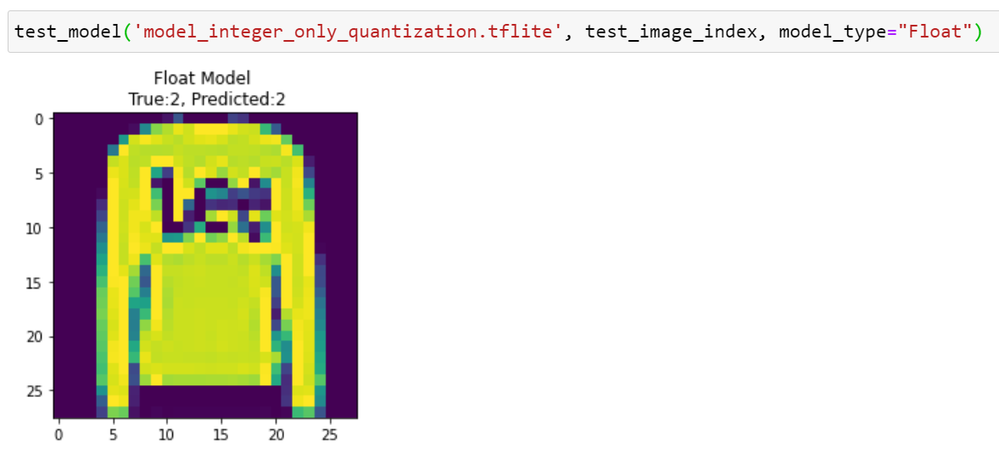Fig 9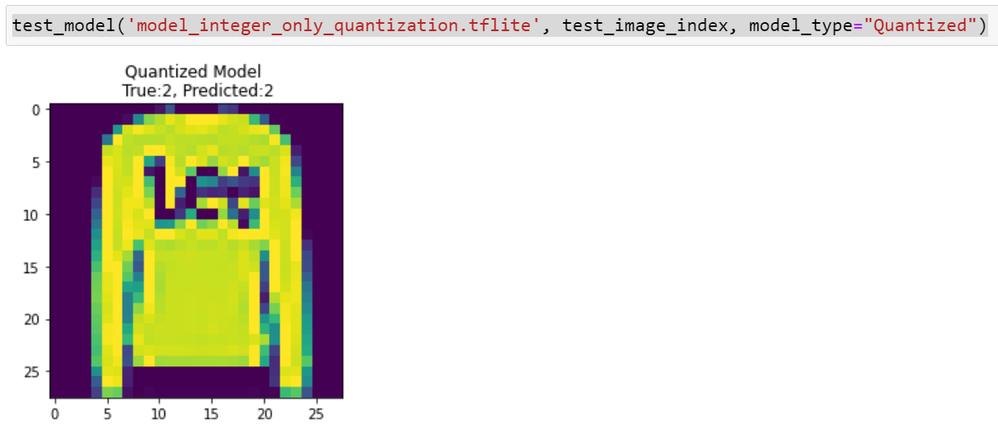Fig 10

Then evaluate the quantized model by using all the test images we loaded at the beginning of this tutorial.
After summarizing the prediction result of the test dataset, we can see that the prediction accuracy of the quantized model decrease 7% less than the original model, it's not bad.

``````# Helper function to evaluate a TFLite model on all images
def evaluate_model(tflite_file, model_type):

test_image_indices = range(test_images.shape)
predictions = run_tflite_model(tflite_file, test_image_indices)

accuracy = (np.sum(test_labels== predictions) * 100) / len(test_images)

print('%s model accuracy is %.4f%% (Number of test samples=%d)' % (
model_type, accuracy, len(test_images)))``````

# Deploying model

• Converting TensorFlow Lite model to C file

The following code runs xxd on the quantized model, writes the output to a file called model_quantized.cc, in the file, the model is defined as an array of bytes, and prints it to the screen. The output is very long, so we won’t reproduce it all here, but here’s a snippet that includes just the beginning and end.

``````# Save the file as a C source file
xxd -i model_integer_only_quantization.tflite > model_quantized.cc
# Print the source file
cat model_quantized.cc``````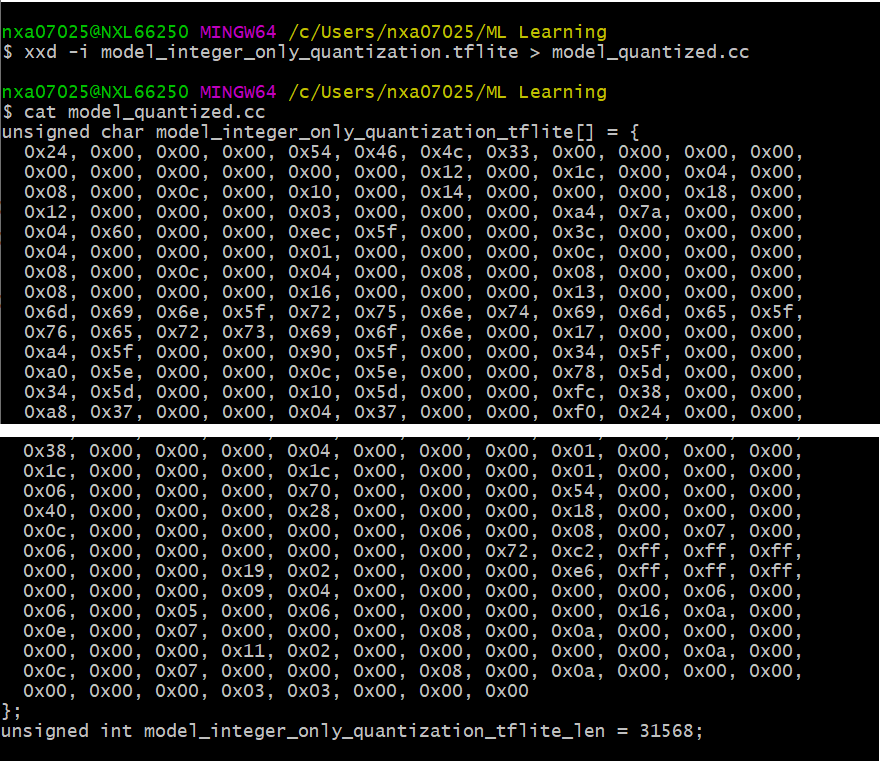Fig 11

• Deploying the C file to project

We use the tensorflow_lite_cifar10 demo as a prototype, then replace the original model and do some code modification, below is the code in the modified main file.

``````#include "board.h"
#include "fsl_debug_console.h"
#include "pin_mux.h"

#include "timer.h"

#include <iomanip>
#include <iostream>
#include <string>
#include <vector>

#include "tensorflow/lite/kernels/register.h"
#include "tensorflow/lite/model.h"
#include "tensorflow/lite/optional_debug_tools.h"
#include "tensorflow/lite/string_util.h"

#include "get_top_n.h"
#include "model.h"

#define LOG(x) std::cout

// ---------------------------- Application -----------------------------
// Lenet Mnist model input data size (bytes).
#define LENET_MNIST_INPUT_SIZE    28*28*sizeof(char)

// Lenet Mnist model number of output classes.
#define LENET_MNIST_OUTPUT_CLASS  10

// Allocate buffer for input data. This buffer contains the input image
// pre-processed and serialized as text to include here.
uint8_t imageData[LENET_MNIST_INPUT_SIZE] = {
#include "clothes_select.inc"
};

/* Tresholds */
#define DETECTION_TRESHOLD 60

/*!
* @brief Initialize parameters for inference
*
* @param reference to flat buffer
* @param reference to interpreter
* @param pointer to storing input tensor address
* @param verbose mode flag. Set true for verbose mode
*/
void InferenceInit(std::unique_ptr<tflite::FlatBufferModel> &model, std::unique_ptr<tflite::Interpreter> &interpreter, TfLiteTensor** input_tensor, bool isVerbose)
{
model = tflite::FlatBufferModel::BuildFromBuffer(Fashion_MNIST_model, Fashion_MNIST_model_len);

if (!model)
{
LOG(FATAL) << "Failed to load model\r\n";
return;
}

tflite::ops::builtin::BuiltinOpResolver resolver;

tflite::InterpreterBuilder(*model, resolver)(&interpreter);
if (!interpreter)
{
LOG(FATAL) << "Failed to construct interpreter\r\n";
return;
}

int input = interpreter->inputs();

const std::vector<int> inputs = interpreter->inputs();
const std::vector<int> outputs = interpreter->outputs();

if (interpreter->AllocateTensors() != kTfLiteOk)
{
LOG(FATAL) << "Failed to allocate tensors!";
return;
}

/* Get input dimension from the input tensor metadata
assuming one input only */
*input_tensor = interpreter->tensor(input);
auto data_type = (*input_tensor)->type;

if (isVerbose)
{
const std::vector<int> inputs = interpreter->inputs();
const std::vector<int> outputs = interpreter->outputs();

LOG(INFO) << "input: " << inputs << "\r\n";
LOG(INFO) << "number of inputs: " << inputs.size() << "\r\n";
LOG(INFO) << "number of outputs: " << outputs.size() << "\r\n";

LOG(INFO) << "tensors size: " << interpreter->tensors_size() << "\r\n";
LOG(INFO) << "nodes size: " << interpreter->nodes_size() << "\r\n";
LOG(INFO) << "inputs: " << interpreter->inputs().size() << "\r\n";
LOG(INFO) << "input(0) name: " << interpreter->GetInputName(0) << "\r\n";

int t_size = interpreter->tensors_size();
for (int i = 0; i < t_size; i++)
{
if (interpreter->tensor(i)->name)
{
LOG(INFO) << i << ": " << interpreter->tensor(i)->name << ", "
<< interpreter->tensor(i)->bytes << ", "
<< interpreter->tensor(i)->type << ", "
<< interpreter->tensor(i)->params.scale << ", "
<< interpreter->tensor(i)->params.zero_point << "\r\n";
}
}

LOG(INFO) << "\r\n";
}
}

/*!
* @brief Runs inference input buffer and print result to console
*
* @param pointer to image data
* @param image data length
* @param pointer to labels string array
* @param reference to flat buffer model
* @param reference to interpreter
* @param pointer to input tensor
*/
void RunInference(const uint8_t* image, size_t image_len, const std::string* labels,
std::unique_ptr<tflite::FlatBufferModel> &model,
std::unique_ptr<tflite::Interpreter> &interpreter,
TfLiteTensor* input_tensor)
{
/* Copy image to tensor. */
memcpy(input_tensor->data.uint8, image, image_len);

/* Do inference on static image in first loop. */
auto start = GetTimeInUS();

if (interpreter->Invoke() != kTfLiteOk)
{
LOG(FATAL) << "Failed to invoke tflite!\r\n";
return;
}

auto end = GetTimeInUS();
const float threshold = (float)DETECTION_TRESHOLD /100;

std::vector<std::pair<float, int>> top_results;
int output = interpreter->outputs();
TfLiteTensor *output_tensor = interpreter->tensor(output);
TfLiteIntArray* output_dims = output_tensor->dims;
// assume output dims to be something like (1, 1, ... , size)
auto output_size = output_dims->data[output_dims->size - 1];

/* Find best image candidates. */
GetTopN<uint8_t>(interpreter->typed_output_tensor<uint8_t>(0), output_size,
1, threshold, &top_results, false);

if (!top_results.empty())
{
auto result = top_results.front();
const float confidence = result.first;
const int index = result.second;
if (confidence * 100 > DETECTION_TRESHOLD)
{
LOG(INFO) << "----------------------------------------\r\n";
LOG(INFO) << "     Inference time: " << (end - start) / 1000 << " ms\r\n";
LOG(INFO) << "     Detected: " << std::setw(10) << labels[index] << " (" << (int)(confidence * 100) << "%)\r\n";
LOG(INFO) << "----------------------------------------\r\n\r\n";
}
}
}

/*!
* @brief Main function
*/
int main(void)
{
const std::string labels[] = {"T-shirt/top", "Trouser","Pullover", "Dress",
"Coat", "Sandal", "Shirt", "Sneaker", "Bag", "Ankle boot"};

/* Init board hardware. */
BOARD_ConfigMPU();
BOARD_InitPins();
BOARD_BootClockRUN();
BOARD_InitDebugConsole();

InitTimer();

std::unique_ptr<tflite::FlatBufferModel> model;
std::unique_ptr<tflite::Interpreter> interpreter;
TfLiteTensor* input_tensor = 0;
InferenceInit(model, interpreter, &input_tensor, false);

LOG(INFO) << "Fashion MNIST object recognition example using a TensorFlow Lite model.\r\n";
LOG(INFO) << "Detection threshold: " << DETECTION_TRESHOLD << "%\r\n";

/* Run inference on static ship image. */
LOG(INFO) << "\r\nStatic data processing:\r\n";
RunInference((uint8_t*)imageData, (size_t)LENET_MNIST_INPUT_SIZE, labels, model, interpreter, input_tensor);
while(1) {}

}``````
• Testing result

After deploying the model in the demo project, then we'll run this demo on the MIMXRT1060 (Fig 12) board for testing.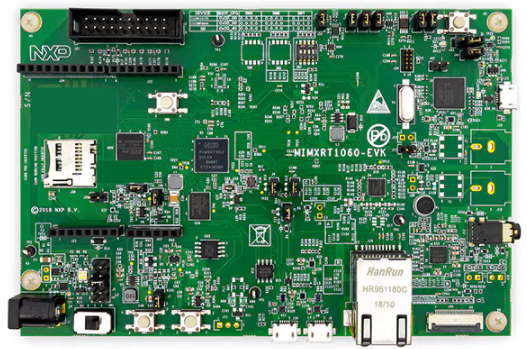Fig 12

1. Run the below code to covert the Fashion MNIST image to text
The process_image() function can convert a Fashion MNIST image to an include file as static data, then include this file in the demo project.
``````def process_image(image, output_path, num_batch=1):
img_data = np.transpose(image, (2, 0, 1))
# Repeat image for batch processing (resulting tensor is NCHW or NHWC)
img_data = np.reshape(img_data, (num_batch, img_data.shape, img_data.shape, img_data.shape))
img_data = np.repeat(img_data, num_batch, axis=0)
img_data = np.reshape(img_data, (num_batch, img_data.shape, img_data.shape, img_data.shape))
# Serialize image batch
img_data_bytes = bytearray(img_data.tobytes(order='C'))
image_bytes_per_line = 20
with open(output_path, 'wt') as f:
idx = 0
for byte in img_data_bytes:
f.write('0X%02X, ' % byte)
if idx % image_bytes_per_line == (image_bytes_per_line - 1):
f.write('\n')
idx = idx + 1
# Return serialized image size
return len(img_data_bytes) ``````

2. Run the demo project on board.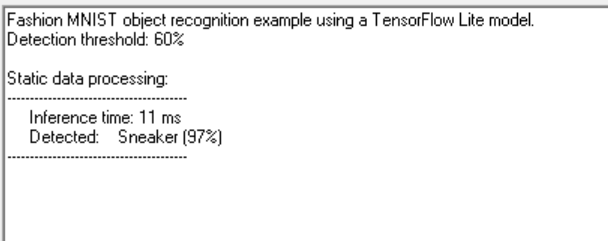Labels (1)
• ### i.MXRT 106x

Tags (3)
Version history
Revision #:
2 of 2
Last update:
‎10-10-2020 12:59 AM
Updated by:Contributors